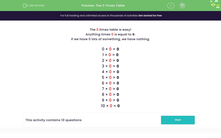# The 0 Times Table

In this worksheet, students answer questions on the 0 times table!Key stage:  KS 1

Curriculum topic:   Number: Multiplication and Division

Curriculum subtopic:   Solve Multiplication/Division Problems

Popular topics:   Times Table worksheets

Difficulty level:#### Worksheet Overview

The 0 times table is easy!

Anything times 0 is equal to 0.

If we have 0 lots of something, we have nothing.

0 × 0 = 0

1 × 0 = 0

2 × 0 = 0

3 × 0 = 0

4 × 0 = 0

5 × 0 = 0

6 × 0 = 0

7 × 0 = 0

8 × 0 = 0

9 × 0 = 0

10 × 0 = 0

### What is EdPlace?

We're your National Curriculum aligned online education content provider helping each child succeed in English, maths and science from year 1 to GCSE. With an EdPlace account you’ll be able to track and measure progress, helping each child achieve their best. We build confidence and attainment by personalising each child’s learning at a level that suits them.

Get started